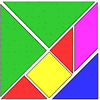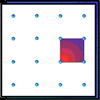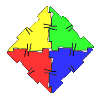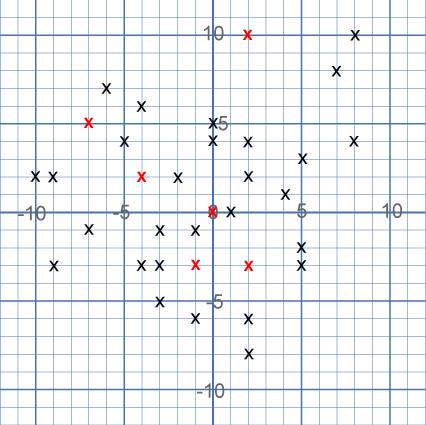#### You may also like### Tangrams

Can you make five differently sized squares from the interactive tangram pieces?### Geoboards

This practical challenge invites you to investigate the different squares you can make on a square geoboard or pegboard.### Polydron

This activity investigates how you might make squares and pentominoes from Polydron.

# Ten Hidden Squares

## Ten Hidden Squares

On the graph below there are $34$ marked points.These points all mark the vertices (corners) of ten hidden squares. Each of the $6$ red points is a vertex shared by two squares. The other $28$ points are each a vertex of just one square. All of the squares share at least one vertex with another square.

All the squares are different sizes.
There are no marked points on the sides of any square, only at the vertices. (There are two near misses!)

Can you find the ten hidden squares?

You might like to play the game Square It which uses some similar ideas.

### Why do this problem?

This problem has two aspects. A knowledge of coordinates in all four quadrants is necessary but also a secure understanding of the properties of squares. The activity challenges learners' misconceptions about how shapes "should be" oriented.

### Possible approach

You could start by drawing some shapes by giving their coordinates, using all four quadrants. This sheet could be used on an interactive whiteboard or enlarged to A3 and drawn on directly. It would be advisable to have one or two squares which are at an angle to the grid to help with the activity itself.

You could continue by choosing one point on the grid of the actual problem and then selecting a second point to form a second corner of a square. You could then ask where a third corner could be now and if there isn't a cross in the position, ask the class to suggest alternatives. Once you have found a possible third corner, ask where the fourth corner would have to be in order to make a square.

After this introduction, the group could work on the rest of the problem with learners working in pairs so that they are able to talk through their ideas with a partner. They could use this sheet for both rough work and for recording. At the end of the lesson it would be useful to discuss where on the grid the $10$ hidden squares are located as well as their different orientations and sizes.

### Key questions

How does that square lie on the grid?
Where could its other vertices be?
Can you find another vertex for it?

### Possible extension

Those who were able to do the problem quickly could plot more squares on this sheet, making sure that all the squares are still different sizes.

### Possible support

Those who are experiencing difficulties could try this simpler version of the problem which only uses coordinates in the first quadrant.